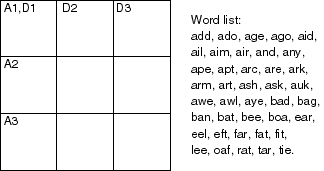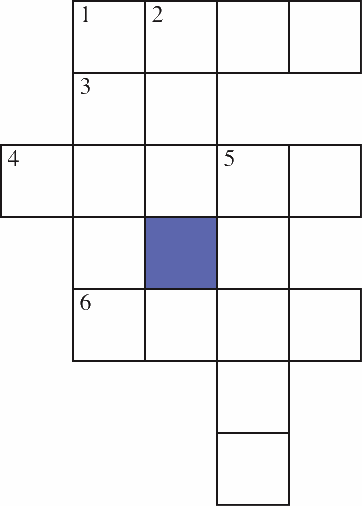## 4.13 Exercises

Exercise 4.1:
Consider the crossword puzzle shown in Figure 4.13.Figure 4.13: A crossword puzzle to be solved with six words

You must find six three-letter words: three words read across (A1, A2, and A3) and three words read down (D1, D2, and D3). Each word must be chosen from the list of forty possible words shown. Try to solve it yourself, first by intuition, then by hand using first domain consistency and then arc consistency.

There are at least two ways to represent the crossword puzzle shown in Figure 4.13 as a constraint satisfaction problem.

The first is to represent the word positions (A1, A2, A3, D1, D2, and D3) as variables, with the set of words as possible values. The constraints are that the letter is the same where the words intersect.

The second is to represent the nine squares as variables. The domain of each variable is the set of letters of the alphabet, {a,b,...,z}. The constraints are that there is a word in the word list that contains the corresponding letters. For example, the top-left square and the center-top square cannot both have the value a, because there is no word starting with aa.

1. Give an example of pruning due to domain consistency using the first representation (if one exists).
2. Give an example of pruning due to arc consistency using the first representation (if one exists).
3. Are domain consistency plus arc consistency adequate to solve this problem using the first representation? Explain.
4. Give an example of pruning due to domain consistency using the second representation (if one exists).
5. Give an example of pruning due to arc consistency using the second representation (if one exists).
6. Are domain consistency plus arc consistency adequate to solve this problem using the second representation?
7. Which representation leads to a more efficient solution using consistency-based techniques? Give the evidence on which you are basing your answer.
Exercise 4.2:
Suppose you have a relation v(N,W) that is true if there is a vowel (one of: a, e, i, o, u) as the N-th letter of word W. For example, v(2,cat) is true because there is a vowel ("a") as the second letter of the word "cat";v(3,cat) is false, because the third letter of "cat" is "t", which is not a vowel; and v(5,cat) is also false because there is no fifth letter in "cat".

Suppose the domain of N is {1,3,5} and the domain of W is {added, blue, fever, green, stare}.

1. Is the arc ⟨N,v⟩ arc consistent? If so, explain why. If not, show what element(s) can be removed from a domain to make it arc consistent.
2. Is the arc ⟨W,v⟩ arc consistent? If so, explain why. If not, show what element(s) can be removed from a domain to make it arc consistent.
Exercise 4.3:
Consider the crossword puzzle shown in Figure 4.14.Figure 4.14: A crossword puzzle to be solved with seven words

The available words that can be used are

at, eta, be, hat, he, her, it, him, on, one, desk, dance, usage, easy, dove, first, else, loses, fuels, help, haste, given, kind, sense, soon, sound, this, think.
1. Given the representation with nodes for the positions (1-across, 2-down, etc.) and words for the domains, specify the network after domain consistency and arc consistency have halted.
2. Consider the dual representation, in which the squares on the intersection of words are the variables and their domains are the letters that could go in these positions. Give the domains after this network has been made arc consistent. Does the result after arc consistency in this representation correspond to the result in part (a)?
3. Show how variable elimination can be used to solve the crossword problem. Start from the arc-consistent network from part (a).
4. Does a different elimination ordering affect the efficiency? Explain.
Exercise 4.4:
Consider how stochastic local search can solve Exercise 4.3. You should use the "stochastic local search" AISpace.org applet to answer this question. Start with the arc-consistent network.
1. How well does random walking work?
2. How well does hill climbing work?
3. How well does the combination work?
4. Give a set of parameter settings that works best.
Exercise 4.5:
Consider a scheduling problem, where there are five activities to be scheduled in four time slots. Suppose we represent the activities by the variables A, B, C, D, and E, where the domain of each variable is {1,2,3,4} and the constraints are A>D, D>E, C ≠A, C>E, C ≠D, B ≥ A, B≠C, and C≠D+1.

[Before you start this, try to find the legal schedule(s) using your own intutions.]

1. Show how backtracking can be used to solve this problem. To do this, you should draw the search tree generated to find all answers. Indicate clearly the valid schedule(s). Make sure you choose a reasonable variable ordering.

To indicate the search tree, write it in text form with each branch on one line. For example, suppose we had variables X, Y, and Z with domains t, f and constraints X ≠Y and Y≠Z. The corresponding search tree can be written as:

```X=t Y=t failure
Y=f Z=t solution
Z=f failure
X=f Y=t Z=t failure
Z=f solution
Y=f failure
```
[

Hint: It may be easier to write a program to generate such a tree for a particular problem than to do it by hand.]

2. Show how arc consistency can be used to solve this problem. To do this you must
• draw the constraint graph;
• show which elements of a domain are deleted at each step, and which arc is responsible for removing the element;
• show explicitly the constraint graph after arc consistency has stopped; and
• show how splitting a domain can be used to sove this problem.
Exercise 4.6:
Which of the following methods can
1. determine that there is no model, if there is not one?
2. find a model if one exists?
3. guarantee to find all models?

The methods to consider are

1. arc consistency with domain splitting.
2. variable elimination.
3. stochastic local search.
4. genetic algorithms.
Exercise 4.7:
Explain how arc consistency with domain splitting can be used to return all of the models and not just one. Give the algorithm.
Exercise 4.8:
Explain how VE can be used to return one of the models rather than all of them. Give the algorithm. How is finding one easier than finding all?
Exercise 4.9:
Explain how arc consistency with domain splitting can be used to count the number of models.
Exercise 4.10:
Explain how VE can be used to count the number of models, without enumerating them all. [Hint: You do not need the backward pass, but instead you can pass forward the number of solutions there would be.]
Exercise 4.11:
Consider the constraint graph of Figure 4.15 with named binary constraints [e.g., r1 is a relation on A and B, which we can write as r1(A,B)]. Consider solving this network using VE.Figure 4.15: Abstract constraint network

1. Suppose you were to eliminate variable A. Which constraints are removed? A constraint is created on which variables? (You can call this r11).
2. Suppose you were to subsequently eliminate B (i.e., after eliminating A). Which relations are removed? A constraint is created on which variables?
Exercise 4.12:
Pose and solve the crypt-arithmetic problem SEND + MORE = MONEY as a CSP. In a crypt-arithmetic problem, each letter represents a different digit, the leftmost digit cannot be zero (because then it would not be there), and the sum must be correct considering each sequence of letters as a base ten numeral. In this example, you know that Y= (D+E) mod 10 and that E= (N+R + ((D+E) ÷10)) mod 10, and so on.